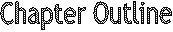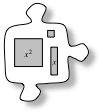# Chapter 6In Section 6.1, you will find ways to divide different amounts of licorice among different numbers of people.  You will gain a deep understanding of division by using diagrams and pictures to model it.  The strategies that you develop in this chapter will help you in Chapter 7 when you will work further with division.

You will begin Section 6.2 by building expressions with a new tool called “algebra tiles.”  You will use variables to describe the perimeters and areas of shapes built with tiles when one dimension is unknown or can represent various lengths. In this chapter, you will learn how to:

• Represent division of fractions using diagrams.
• Divide whole and mixed numbers by fractions.
• Use the order of operations to find the correct value of a numerical expression.
• Combine like terms and simplify algebraic expressions.
• Use a variable to represent any number.Section 6.1    In this section, you will begin by looking at integer division by dividing different amounts of licorice among different numbers of people.  You will use diagrams and other strategies to divide the licorice equally.  Then you will divide fractions and mixed numbers and use your knowledge of division to solve problems.Section 6.2    This section introduces algebra tiles and uses their areas and perimeters to develop the skills of building expressions and combining like terms.  You will simplify and evaluate algebraic expressions for given values.
• Math Notes
• ## Mathematical Vocabulary

• The following is a list of vocabulary found in this chapter.  Some of the words have been seen in a previous chapter.  The italicized words are new to this chapter.  Make sure that you are familiar with the terms below and know what they mean.  Click on the word for a “pop-up” definition. For more information, refer to the glossary or index.  You might also add these words to your Toolkit so that you can reference them in the future.
•  area algebraic expression coefficient combining like terms constant term dimensions equivalent expressions evaluate Order of Operations product quotient ratio rule simplify term variable